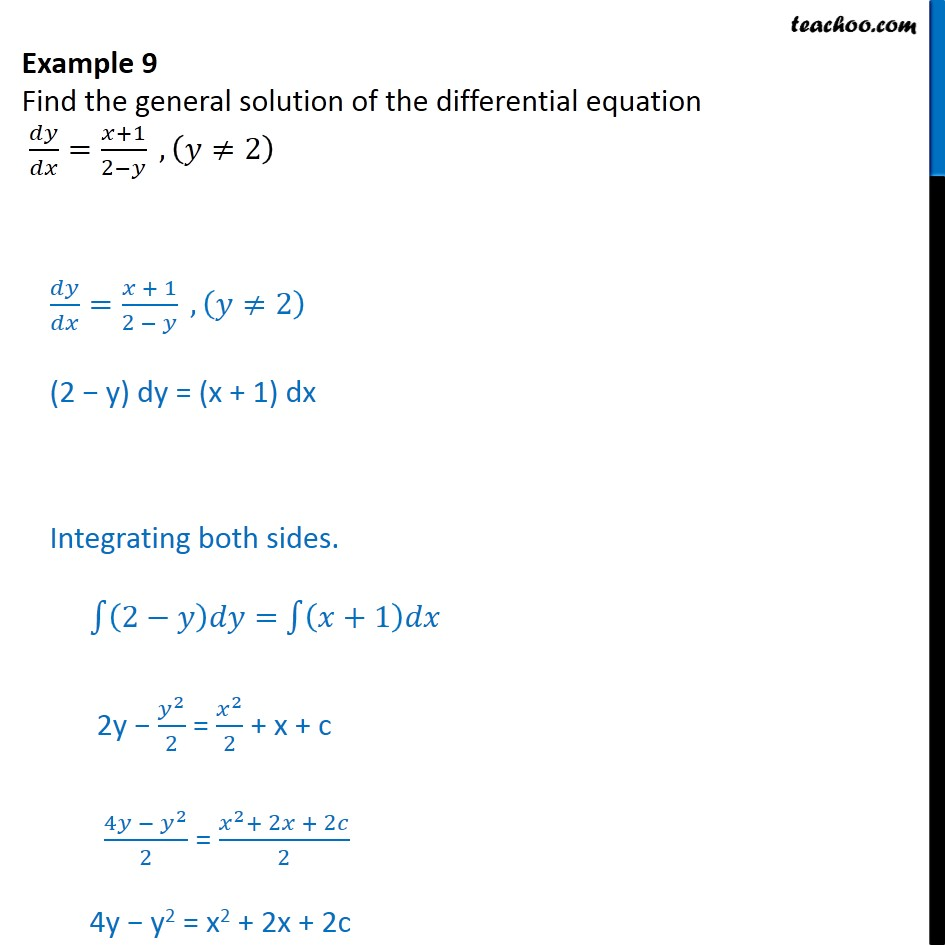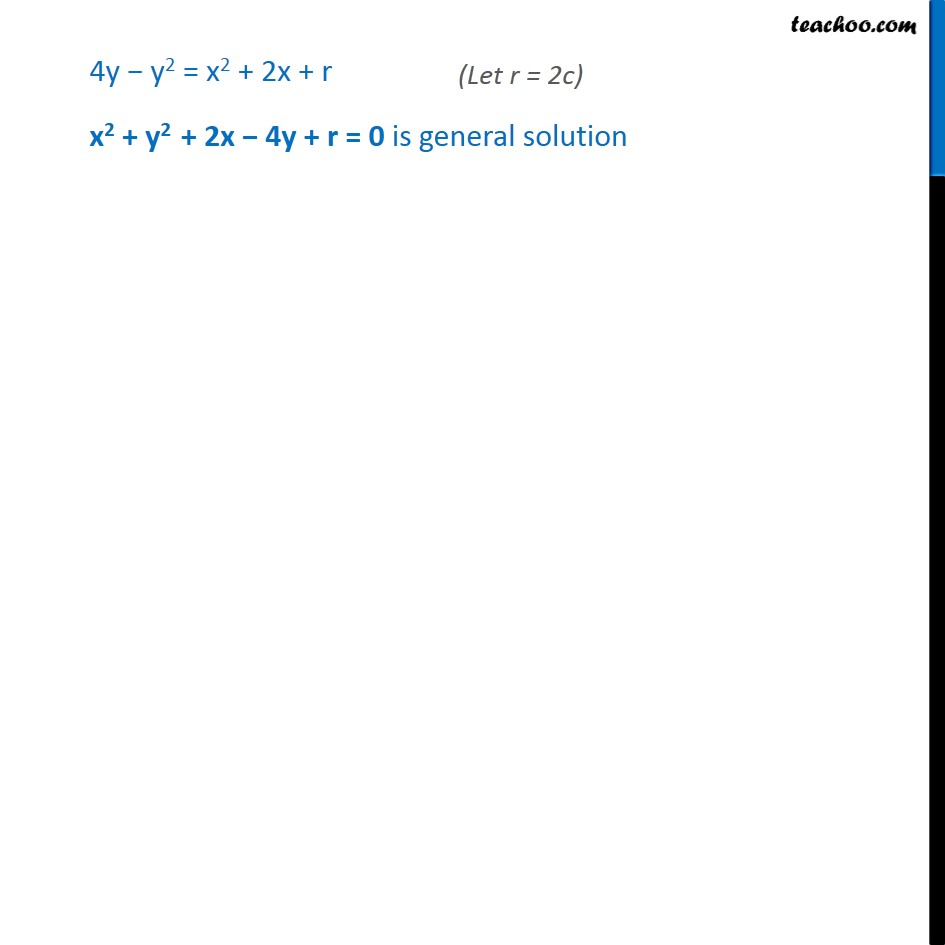Examples

Chapter 9 Class 12 Differential Equations
Serial order wiseLearn in your speed, with individual attention - Teachoo Maths 1-on-1 Class

### Transcript

Example 4 Find the general solution of the differential equation 𝑑𝑦/𝑑𝑥=(𝑥+1)/(2−𝑦) , (𝑦≠2) 𝑑𝑦/𝑑𝑥=(𝑥 + 1)/(2 − 𝑦) , (𝑦≠2) (2 − y) dy = (x + 1) dx Integrating both sides. ∫1▒〖(2−𝑦)𝑑𝑦=〗 ∫1▒(𝑥+1)𝑑𝑥 2y − 𝑦^2/2 = 𝑥^2/2 + x + c 〖4𝑦 − 𝑦〗^2/2 = (𝑥^2+ 2𝑥 + 2𝑐)/2 4y − y2 = x2 + 2x + 2c 4y − y2 = x2 + 2x + r x2 + y2 + 2x − 4y + r = 0 is general solution